# Counting British Coins: Adding Pounds and Pence

Counting British Coins: Adding Pounds and Pence• £1 is worth 100 pence and here are some common ways to make one pound.
• £1 is equivalent to two 50p coins.
• £1 is equivalent to a 50p coin, two 20p coins plus a 10p coin.
• £1 is equivalent to five 20p coins.
• £1 is equivalent to ten 10p coins.
• Any combination of coins that equals 100 pence is equivalent to one pound.

Any combination of coins that add up to 100 pence is worth £1.

#### Here is a downloadable summary sheet showing some of the different ways to make a pound.• We can count money more easily by separating it into pounds and pence.
• We have three pounds made from the £2 plus the £1.
• We have 90p made from the 50p plus the two twenty pence coins.
• We can write the £3 and 90p as £3.90.#### How to Add British Money# Counting Money: Pounds and Pence

## How to Add Pounds and Pence

To add pounds and pence coins use the following steps:

1. Separate the coins into pounds and pence.
2. Add the pound coins together to find the total number of pounds.
3. Gather the pence coins into groups worth 100 pence.
4. Each group of 100 pence is worth 1 pound, which can be added to the pounds total.
5. Count the remaining pence separately.

For example, here is a group of British coins, which we will add to find a total.

The first step is to separate the pound coins from the pence coins.We have a two pound coin and a one pound coin.

2 + 1 = 3 and so £2 + £1 = £3. We have three pounds in pound coins.

We will now count the pence coins.

We have 50p + 20p + 20p.

50 + 20 + 20 = 90 and so, we have 90p.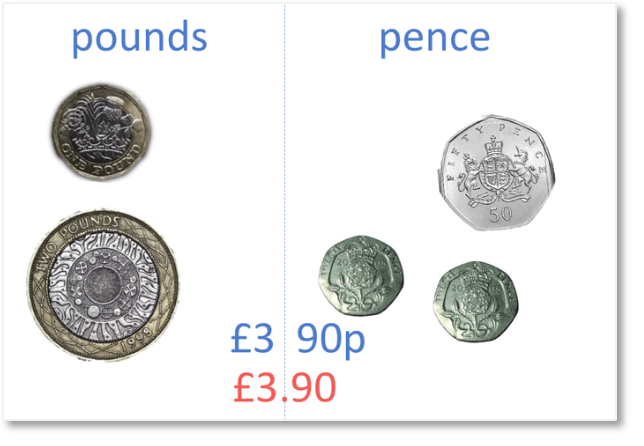In total we have £3 and 90p. We write this as £3.90.

To write pounds and pence, the pounds are written first, before the decimal point and the pence is written after the decimal point. £3 and 90 pence is written as £3.90.Here is another example of counting pounds and pence.

We first separate the pound and pence coins.

We have two one pound coins making a total of £2.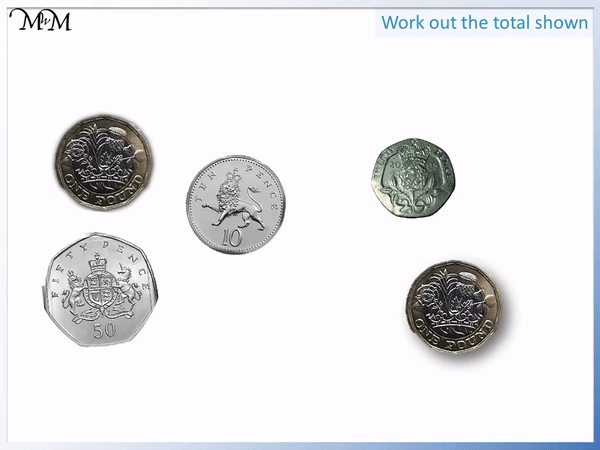Counting the pence coins, we have 50p + 20p + 10p, which equals a total of 80 pence.

In total we have £2 and 80 pence, which is written as £2.80. We separate the pounds and the pence with a decimal point.

## How to Make a Pound with Coins

Here are some examples of how to make a pound using different pence coins:

• 100 × 1p
• 50 × 2p
• 20 × 5p
• 10 × 10p
• 5 × 20p
• 2 × 50p

Any combination of coins that add up to 100 pence are worth £1.

Here are some examples of how to make one pound.We can add combinations of coins by grouping them into totals that equal 100 pence. Each group of 100 pence is worth a pound.

In this example we have a £1 coin along with two 50p coins and two 20p coins.

We have £1 in pounds and we will look for combinations of pence coins that add to 100 pence.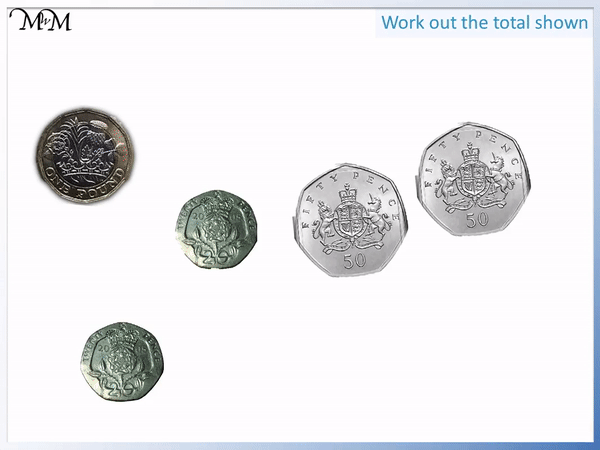We have two 50p coins which both add to make 100p. Two 50p coins are worth £1.

We have £2 in total and two remaining 20p coins. 20p + 20p = 40p.

In total we have 2 pounds and 40 pence. We write this as £2.40. The decimal point separates the pounds and the pence.

Here is another example of finding the total of a combination of coins.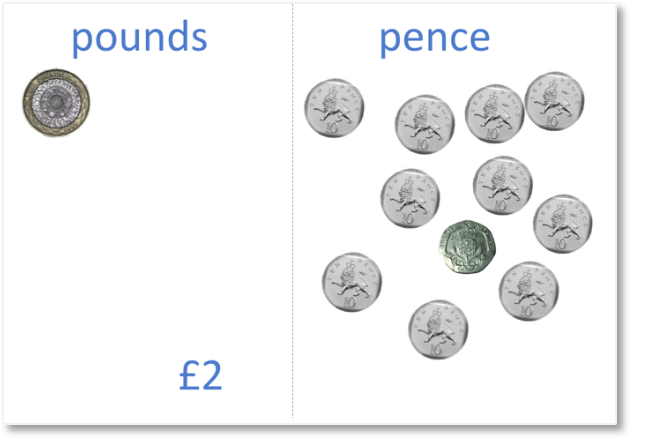We have a £2 coin. We then look for combinations of coins that add together to make a pound.

We can see that we have ten 10p coins. 10 × 10p = 100p. Ten 10p coins are worth £1.In total we have £3 and 20 pence. We write this as £3.20.

Here is another example of adding penny coins.

We do not have any pound coins and so, we first look for groups of penny coins that add to make 100 pence.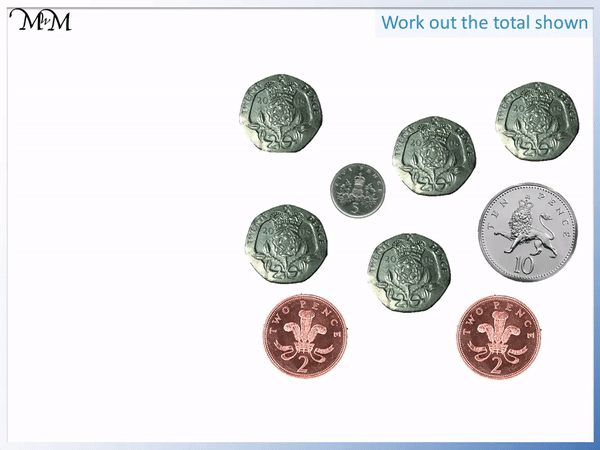We have the combination of five 20p coins. Five times 20p equals 100p and so, five 20p coins are worth £1.

We have £1 and 19 pence, which is written as £1.19.

In this example, we have two pound coins and some pence coins.We look for a combination of pence coins that also add to make one pound.

It is easiest to start by adding the largest value coins. We start with 50p. We can add the two 20p coins to this 50p.

50p + 20p + 20p = 90p. We need 10p more to make 100 pence.

Therefore 50p + 20p + 20p + 10p equals £1.

We move these coins over to the pounds total. There is 10 pence remaining in the form of two 5p coins.

We have £3 and 10 pence, which is written as £3.10.

Sometimes we cannot make exactly one pound because we cannot make a group of exactly 100 pence.

Here is a collection of coins, with £2 in pound coins and some more pence coins.We cannot make 100 pence exactly so we look for a value that makes at least 100 pence.

50p + 20p = 70p.

50p + 20p + 20p = 90p.

50p + 20p + 20p + 20p = 110p.

We will move the 50p and the three 20p coins over to the pounds column. 110p is worth £1 and 10 pence.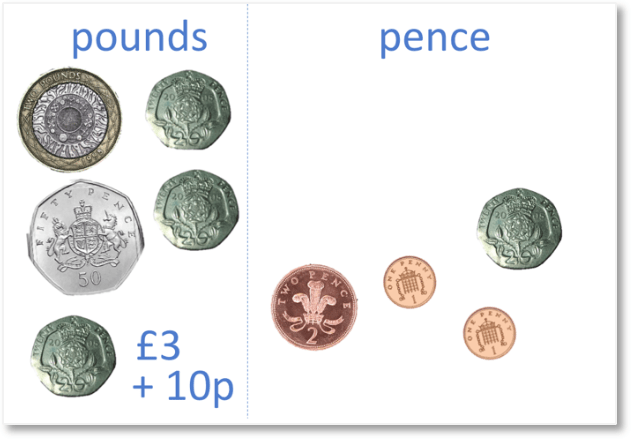In the pounds column, we have £3 and 10 pence. We have 24 pence in the pence column.

In total we have £3 and 34 pence, or £3.34.We can also add pounds and pence by writing the pounds in terms of pence.

Each pound coin can be written as 100 pence and then the totals can be added using column addition.

Here is an example of a collection of coins that will be added together to find a total.

The £1 coin will be written as 100 pence.The total can be found by adding the digits of each coin using column addition as shown below.

In the first column, we have 1 + 2 = 3.

In the next column, we have 2 + 5 = 7.

In the next column, we have 1.

In total, this is £1.73.We have a £2 coin, two 10p coins and a 5p coin.

We write the £2 coin as being worth 200 pence.

We add 200p, 10p, 10p and 5p.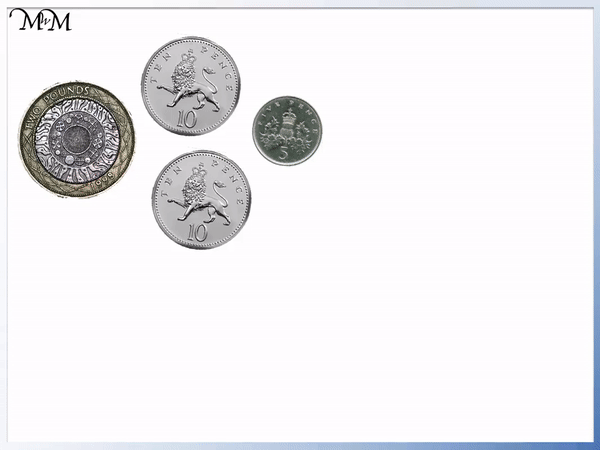In the first column, we have 5.

In the second column we have 1 + 1 = 2.

In the next column, we have 2.

In total we have £2.25.

If the coins can be easily counted into groups worth 100 pence, then it is easiest to group the coins into collections of pounds and add them.

For combinations of coins that cannot be made into pounds, then column addition can be an easier method for adding money.Now try our lesson Reading Hours on a Clock where we learn how to tell the time to the hour.error: Content is protected !!